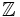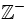# Integer

An integer is any number that does not have any fractional or decimal components. Numbers such as 1,2 and -3 are integers, but 2½, √5 are not. The mathematical symbol for this set is. More precisely, the set of all integers consists of all natural numbers {1, 2, 3, 4, ...}, their negatives {-1, -2, -3, -4, ...} and 0. A formal definition is that it is the only integral domain whose positive elements are well ordered and in which order is preserved by addition. Integers are often colloquially referred to as "whole numbers," though this terminology is not well defined and is often used to refer to only a subset of integers, such as the positive integers.

An integer may be:

Every integer larger than 1 has a unique prime factorization.

Some examples of integers: 1, 10/5, 98058493, -87, -3/3, both square roots of 9, and 0.

Likewise, the following numbers are not integers: 5/10, the square root of -9, 8.75, and pi.

Some subsets of the integers are often used. They have their own symbols:

set name symbol
..., -2, -1, 0, 1, 2, ... integers1, 2, 3, 4, ... positive integers0, 1, 2, 3, 4, ... nonnegative integers0, -1, -2, -3, -4, ... nonpositive integers
-1, -2, -3, -4, ... negative integers